# Limits & Continuity Questions and Answers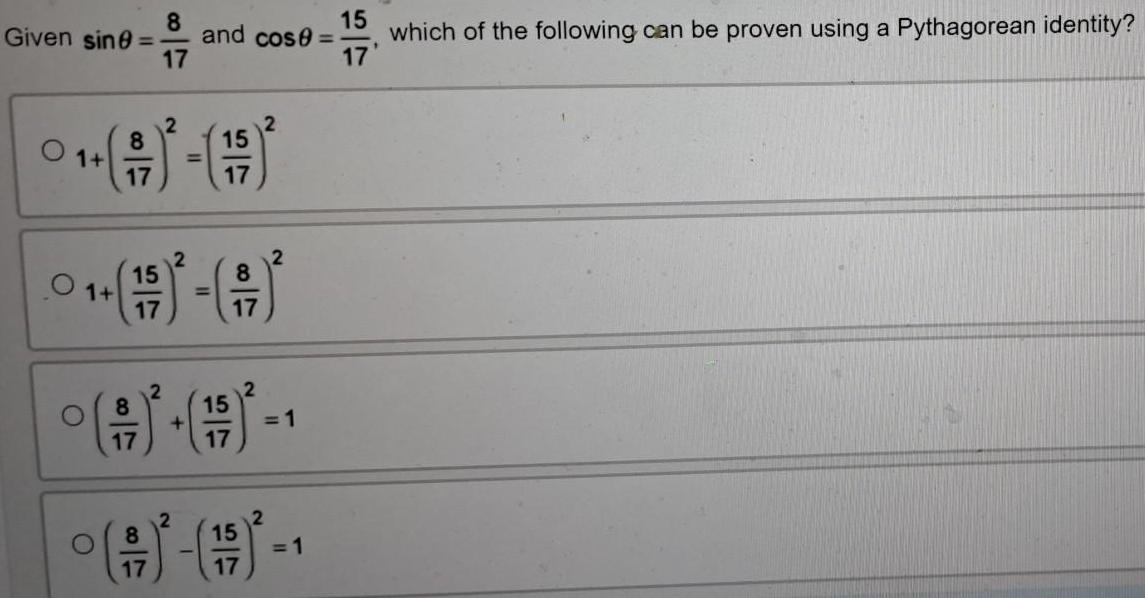Calculus
Limits & Continuity
Given sinθ = 8/17 and cosθ = 15/17 which of the following can be proven using a Pythagorean identity? 1 + (8/17)^2 = (15/17)^2 1 + (15/17)^2 = (8/17)^2 (8/17)^2 + (15/17)^2 = 1 (8/17)^2 - (15/17)^2 = 1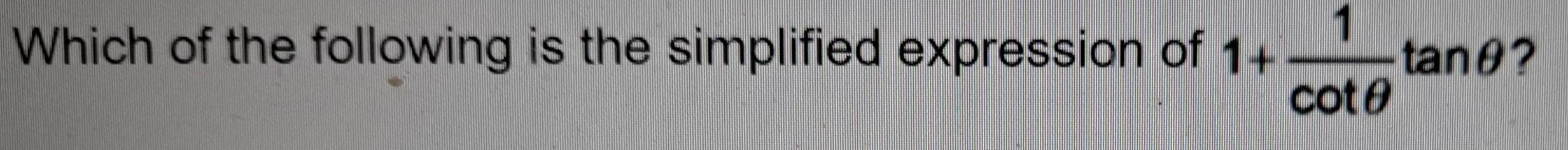Calculus
Limits & Continuity
1 Which of the following is the simplified expression of 1+ 1/cotθ tanθ?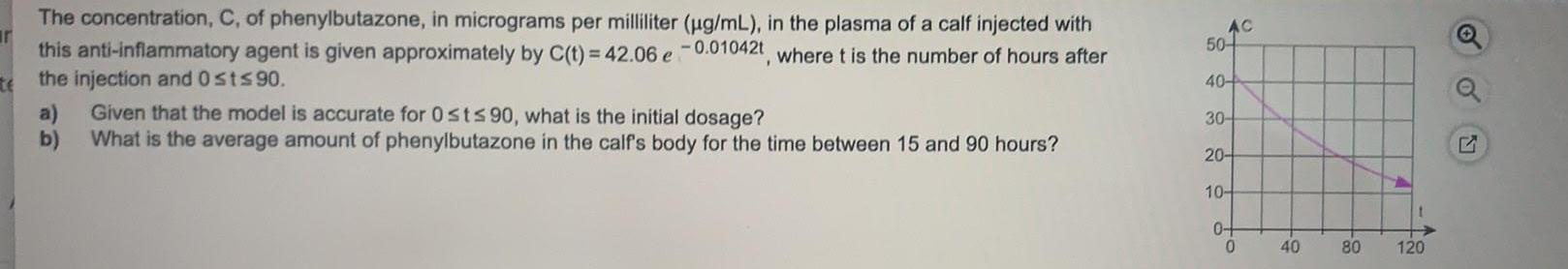Calculus
Limits & Continuity
The concentration, C, of phenylbutazone, in micrograms per milliliter (µg/mL), in the plasma of a calf injected with this anti-inflammatory agent is given approximately by C(t) = 42.06 e^ -0.01042t, where t is the number of hours after the injection and 0 st≤90. a) Given that the model is accurate for 0 st≤ 90, what is the initial dosage? b) What is the average amount of phenylbutazone in the calf's body for the time between 15 and 90 hours?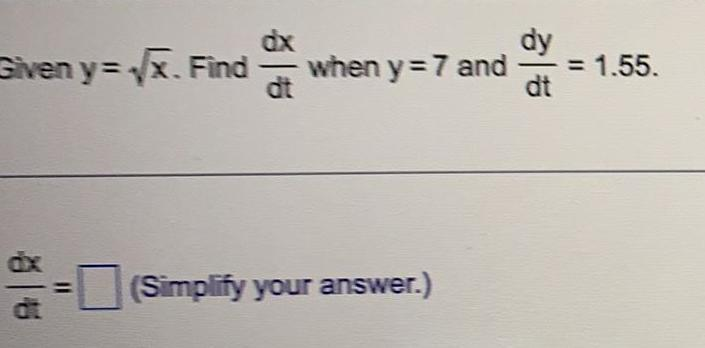Calculus
Limits & Continuity
Given y=√x. Find dx/dt when y=7 and dy/dt = 1.55.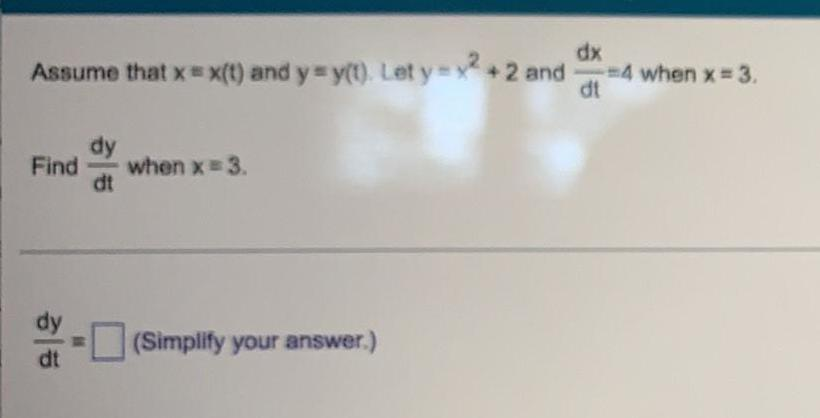Calculus
Limits & Continuity
Assume that x = x(t) and y=y(t). Let y = x² +2 and dx/dt = 4 Find dy/dt when x = 3.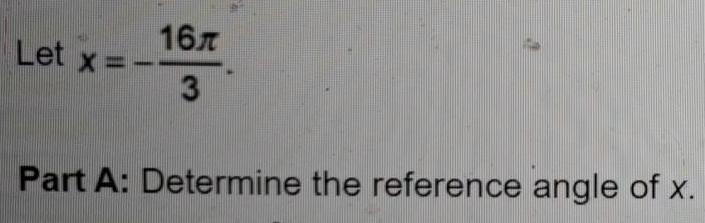Calculus
Limits & Continuity
Let x = -16π/3 Part A: Determine the reference angle of x.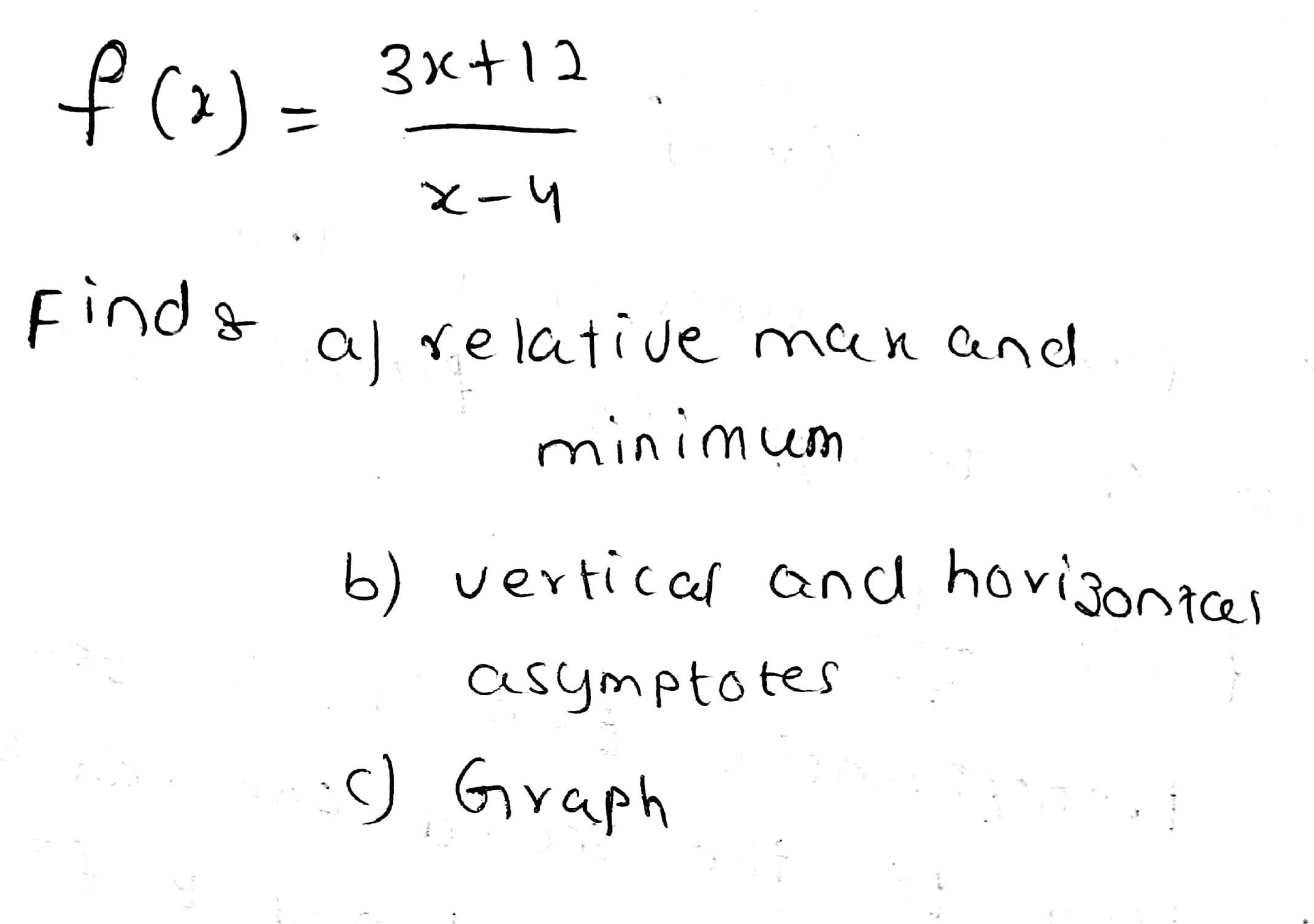Calculus
Limits & Continuity
f(x) = 3x+12/x-4 Finds a. relative maximum and minimum b. vertical and horisontal asymptotes c. Graph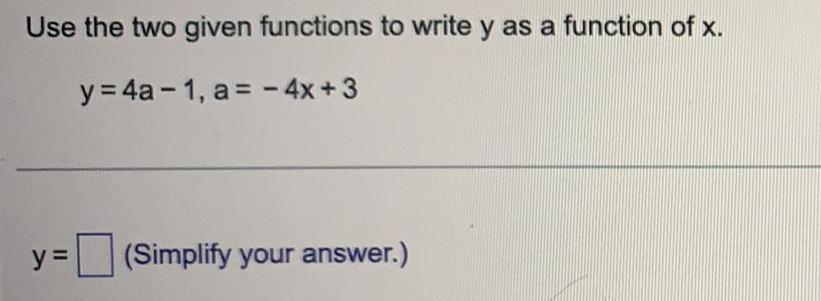Calculus
Limits & Continuity
Use the two given functions to write y as a function of x. y = 4a-1, a = - 4x +3 y = (Simplify your answer.)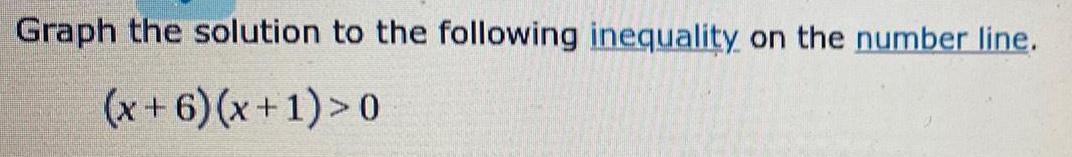Calculus
Limits & Continuity
Graph the solution to the following inequality on the number line. (x+6) (x+1)>0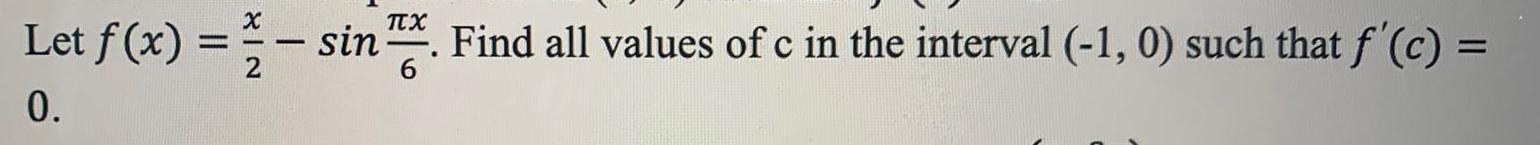Calculus
Limits & Continuity
Let f(x) = x/2 - sin πx/6. Find all values of c in the interval (-1, 0) such that f'(c) =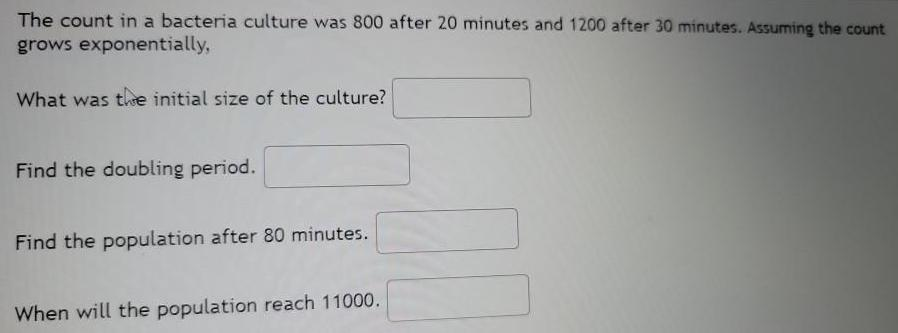Calculus
Limits & Continuity
The count in a bacteria culture was 800 after 20 minutes and 1200 after 30 minutes. Assuming the count grows exponentially, What was the initial size of the culture? Find the doubling period. Find the population after 80 minutes. When will the population reach 11000.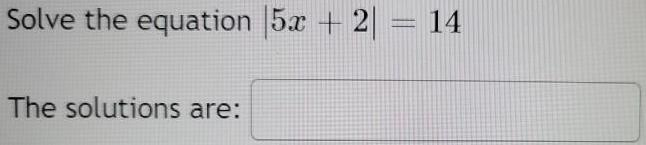Calculus
Limits & Continuity
Solve the equation |5x + 2| = 14 The solutions are: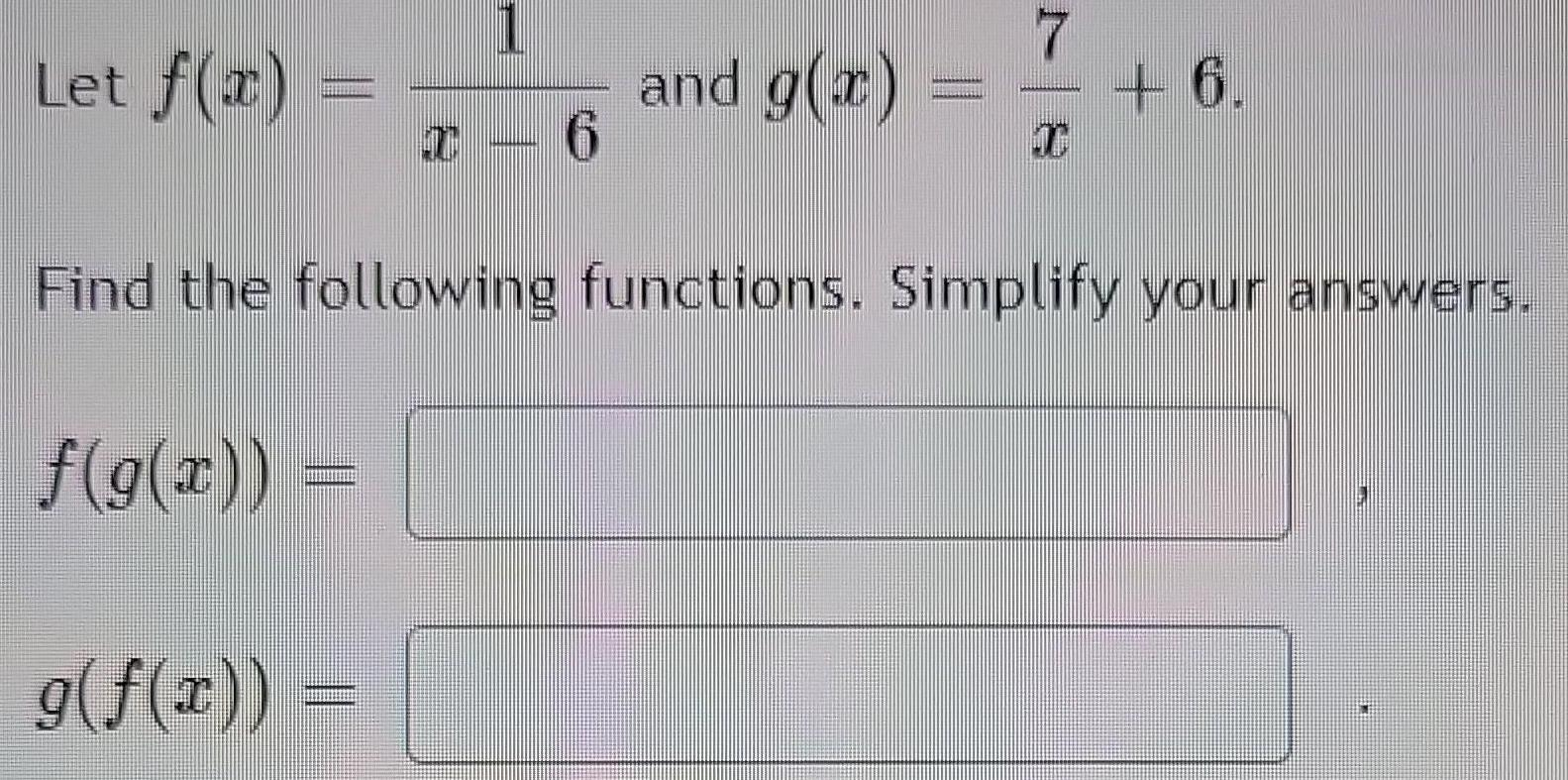Calculus
Limits & Continuity
Let f(x) = 1/x-6 and g(x) = 7/x + 6 Find the following functions. Simplify your answers. f(g(x)) = g(f(x)) =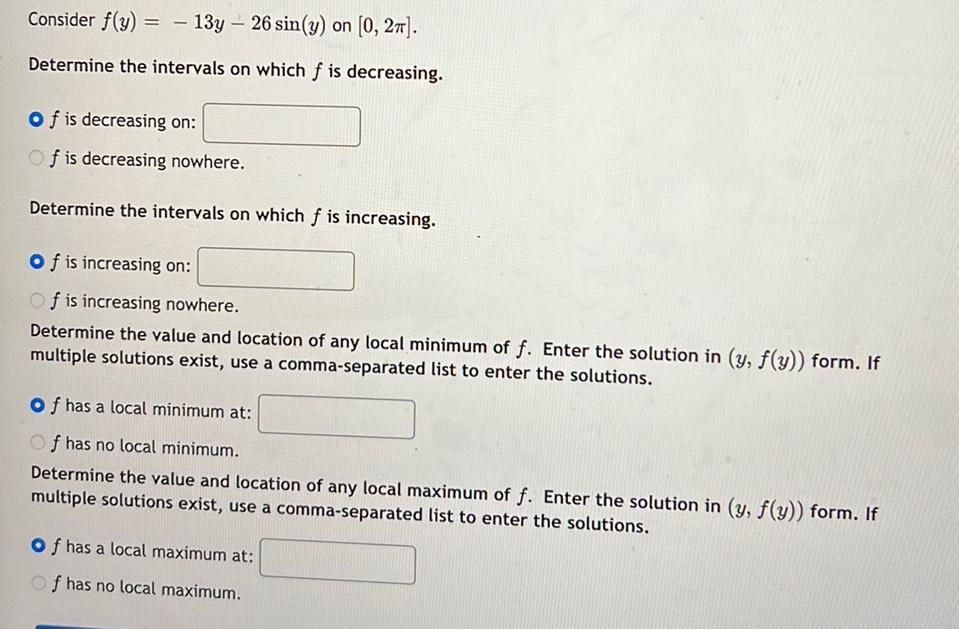Calculus
Limits & Continuity
Consider f(y) = -13y - 26 sin(y) on [0, 2π]. Determine the intervals on which f is decreasing. f is decreasing on: f is decreasing nowhere. Determine the intervals on which f is increasing. f is increasing on: f is increasing nowhere. Determine the value and location of any local minimum of f. Enter the solution in (y, f(y)) form. If multiple solutions exist, use a comma-separated list to enter the solutions. f has a local minimum at: f has no local minimum. Determine the value and location of any local maximum of f. Enter the solution in (y, f(y)) form. If multiple solutions exist, use a comma-separated list to enter the solutions. f has a local maximum at: f has no local maximum.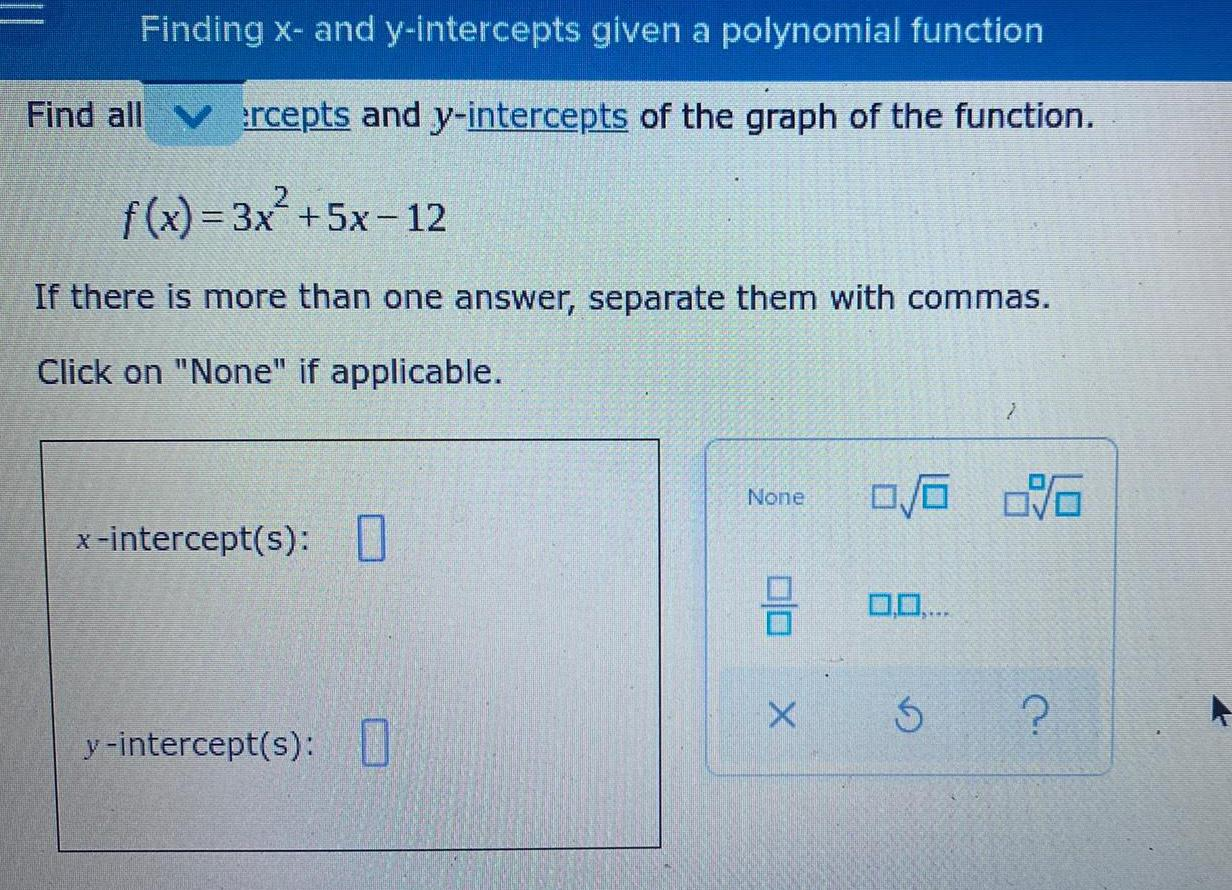Calculus
Limits & Continuity
Finding x- and y-intercepts given a polynomial function Find all ercepts and y-intercepts of the graph of the function. f(x) = 3x² +5x-12 If there is more than one answer, separate them with commas. Click on "None" if applicable.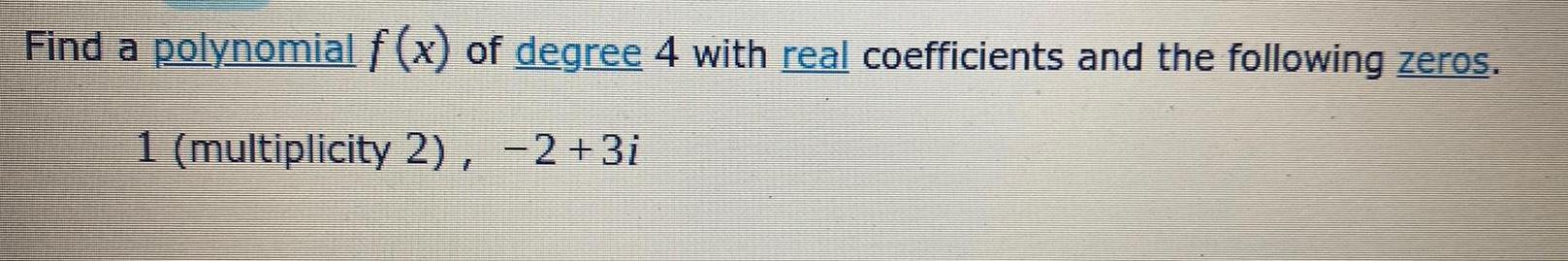Calculus
Limits & Continuity
Find a polynomial f (x) of degree 4 with real coefficients and the following zeros. 1 (multiplicity 2), -2+3i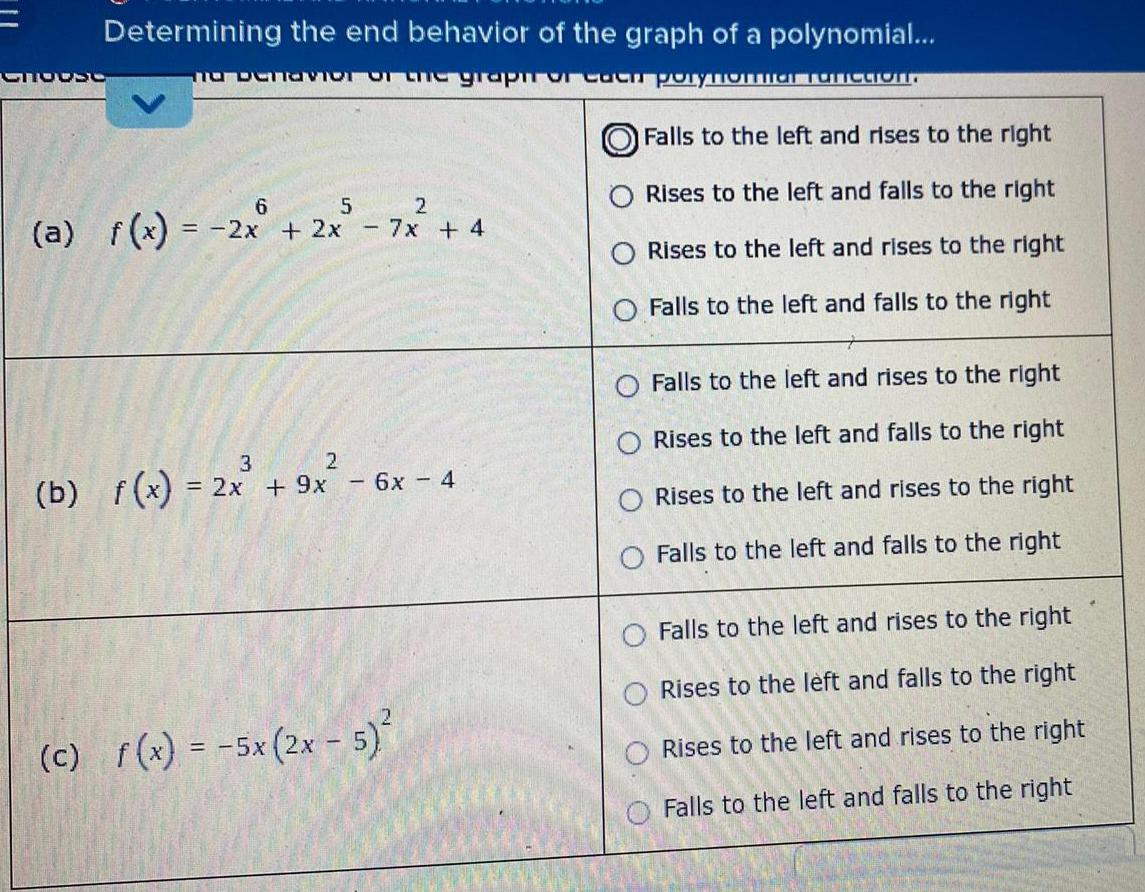Calculus
Limits & Continuity
Determining the end behavior of the graph of a polynomial... (a) f(x)= = -2x + 2x - 7x + 4 Falls to the left and rises to the right Rises to the left and falls to the right Rises to the left and rises to the right Falls to the left and falls to the right (b) f(x) = 2x3 + 9x2 - 6x-4 Falls to the left and rises to the right Rises to the left and falls to the right Rises to the left and rises to the right Falls to the left and falls to the right (c) f(x) = -5x (2x - 5)² Falls to the left and rises to the right Rises to the left and falls to the right Rises to the left and rises to the right Falls to the left and falls to the right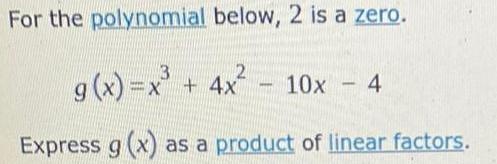Calculus
Limits & Continuity
For the polynomial below, 2 is a zero. g(x)=x² =x² + 4x² Express g (x) as a product of linear factors. www. 10x - 4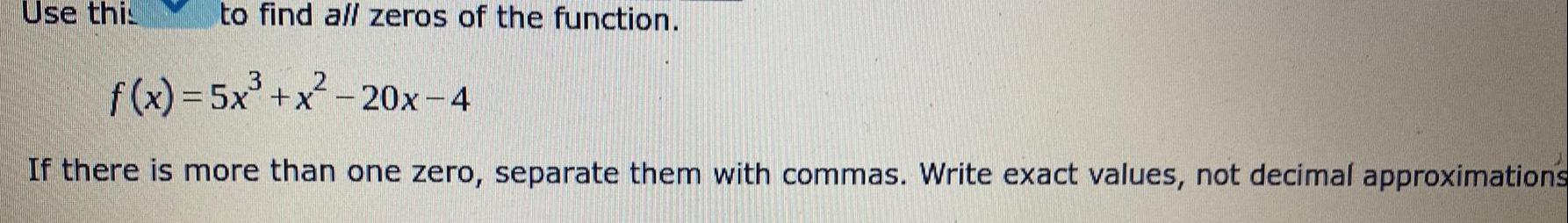Calculus
Limits & Continuity
Use this to find all zeros of the function. f(x) = 5x³+x²2-20x-4 If there is more than one zero, separate them with commas. Write exact values, not decimal approximations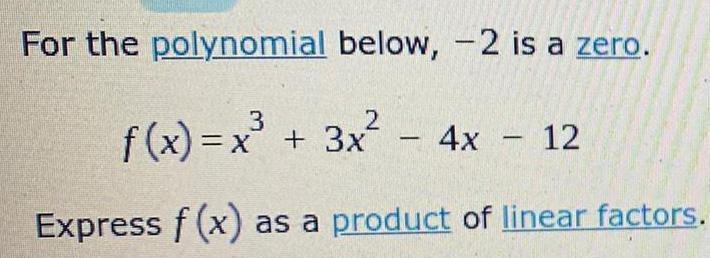Calculus
Limits & Continuity
For the polynomial below, -2 is a zero. f(x)=x² + 3x² - 4x - 12 Express f(x) as a product of linear factors.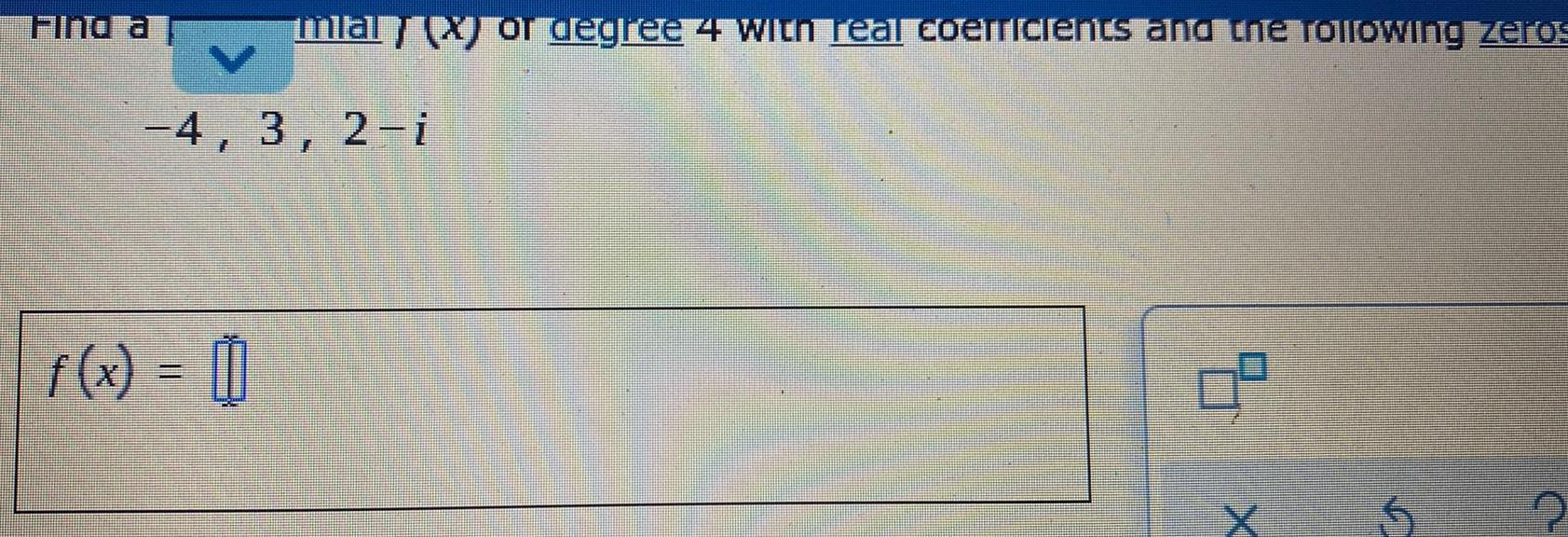Calculus
Limits & Continuity
Find a mla -4, 3, 2-i f(x) = 11 degree 4 with real coerricle and the following zeros 0² x 5 U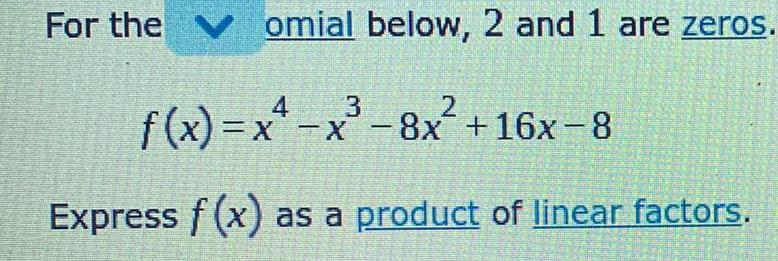Calculus
Limits & Continuity
For the omial below, 2 and 1 are zeros. 4 3 f(x)=x²-x³-8x² +16x-8 Express f(x) as a product of linear factors. 2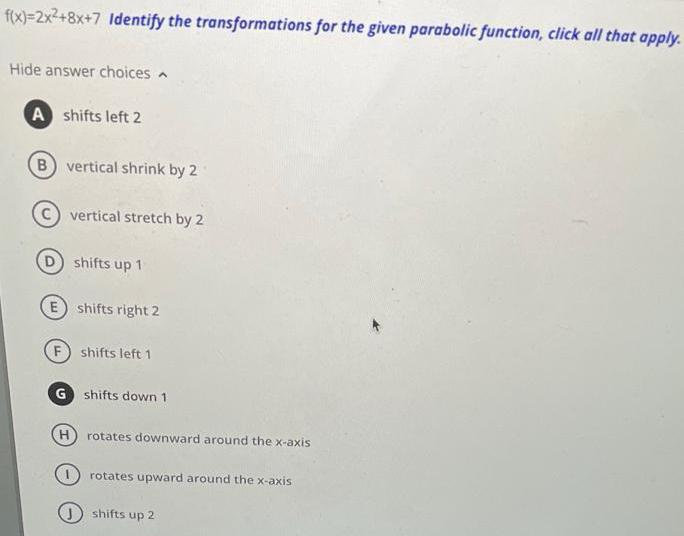Calculus
Limits & Continuity
f(x)=2x²+8x+7 Identify the transformations for the given parabolic function, click all that apply. Hide answer choices A shifts left 2 B) vertical shrink by 2 vertical stretch by 2 D) shifts up 1 E shifts right 2 (F) shifts left 1 G shifts down 1 (H) rotates downward around the x-axis rotates upward around the x-axis shifts up 2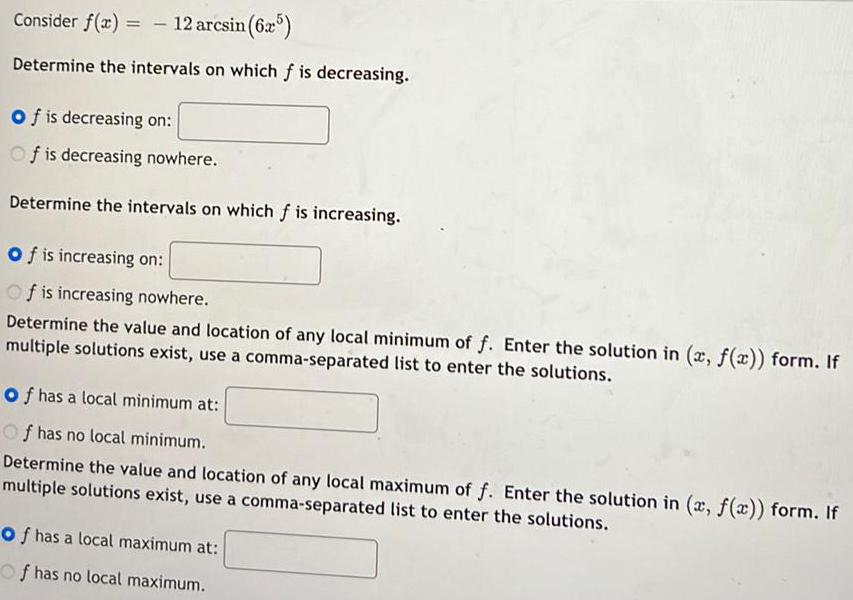Calculus
Limits & Continuity
Consider f(x) = -12 arcsin (625) Determine the intervals on which f is decreasing. Of is decreasing on: Of is decreasing nowhere. Determine the intervals on which f is increasing. Of is increasing on: Of is increasing nowhere. Determine the value and location of any local minimum of f. Enter the solution in (x, f(x)) form. If multiple solutions exist, use a comma-separated list to enter the solutions. of has a local minimum at: Of has no local minimum. Determine the value and location of any local maximum of f. Enter the solution in (x, f(x)) form. If multiple solutions exist, use a comma-separated list to enter the solutions. of has a local maximum at: Of has no local maximum.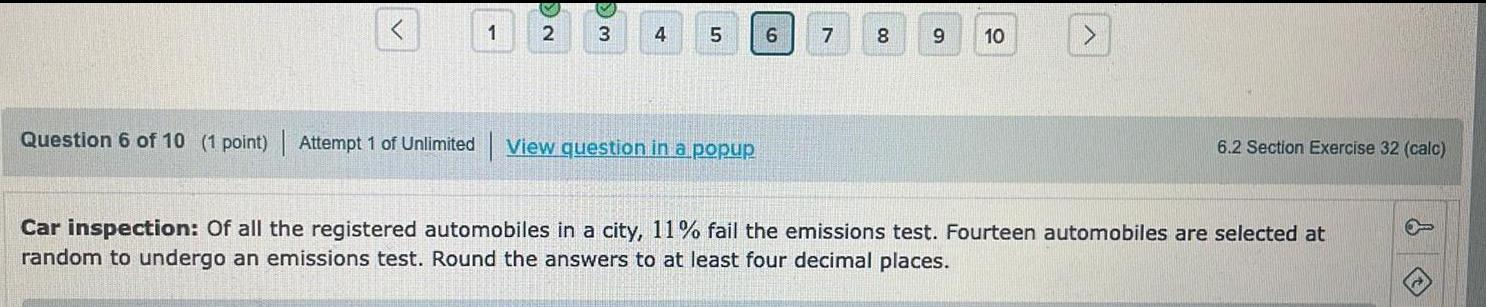Calculus
Limits & Continuity
Question 6 of 10 (1 point) | Attempt 1 of Unlimited View question in a popup Car inspection: Of all the registered automobiles in a city, 11% fail the emissions test. Fourteen automobiles are selected at random to undergo an emissions test. Round the answers to at least four decimal places.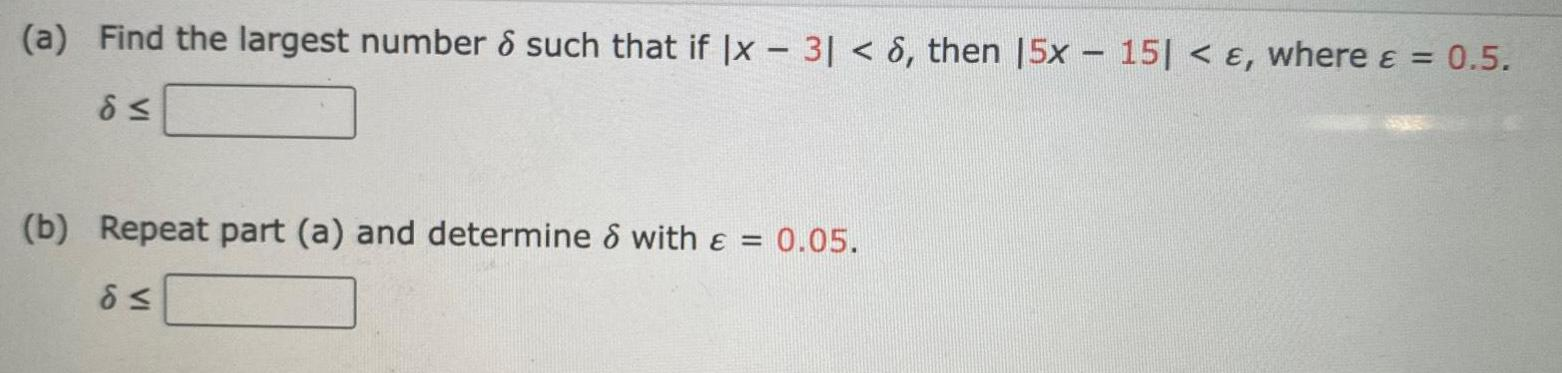Calculus
Limits & Continuity
(a) Find the largest number 6 such that if Ix - 3| < 6, then [5x - 15| < &, where & = 0.5. d≤ (b) Repeat part (a) and determine & with & = 0.05. d≤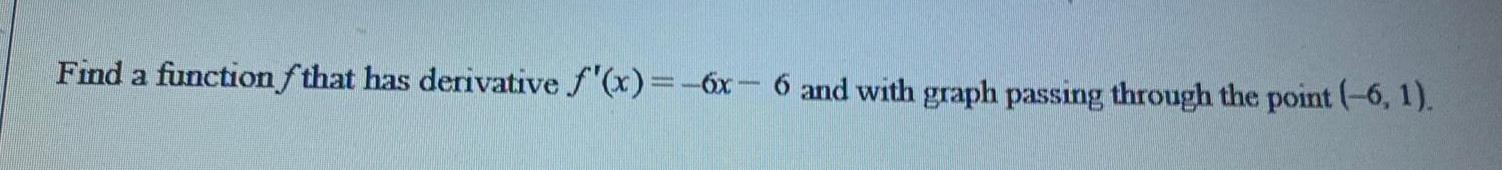Calculus
Limits & Continuity
Find a function that has derivative f'(x) = -6x-6 and with graph passing through the point (-6, 1).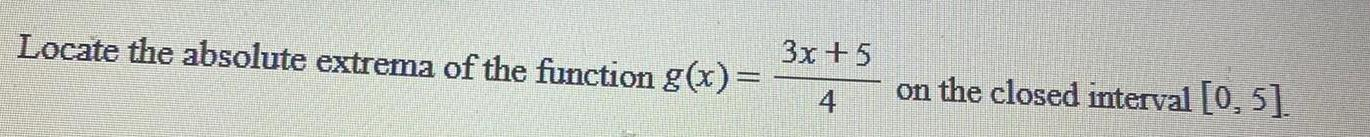Calculus
Limits & Continuity
Locate the absolute extrema of the function g(x)= 3x +5 on the closed interval [0, 5]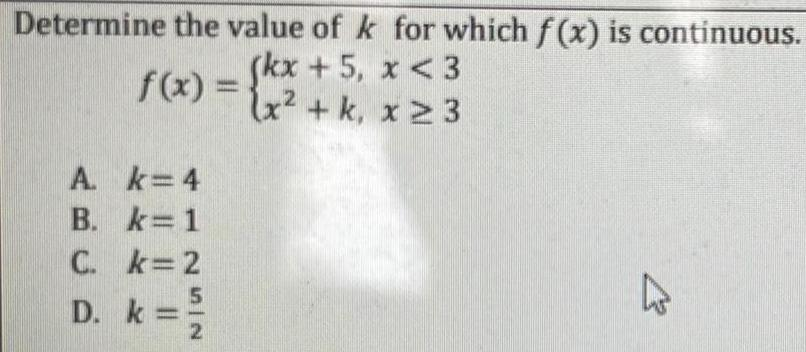Calculus
Limits & Continuity
Determine the value of k 5, f(x) = {kx + 5₁ A k=4 B. k=1 C. k=2 D. k= 5 IN 2 for which f(x) is continuous. x <3 (x² + k, x ≥ 3 K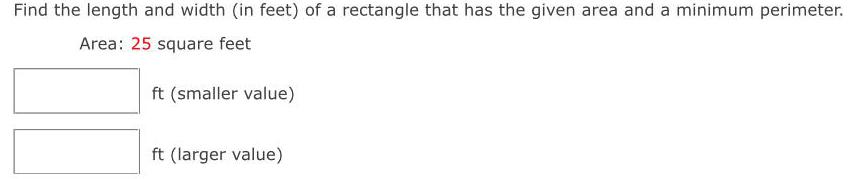Calculus
Limits & Continuity
Find the length and width (in feet) of a rectangle that has the given area and a minimum perimeter. Area: 25 square feet ft (smaller value) ft (larger value)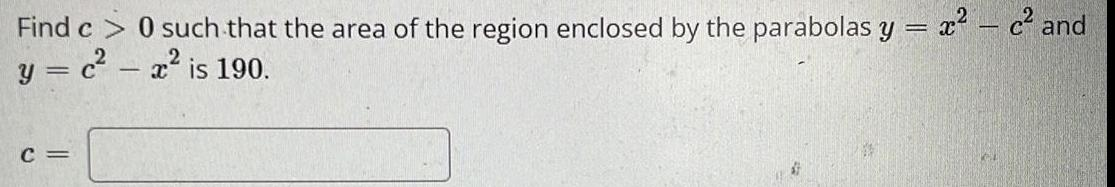Calculus
Limits & Continuity
Find c> 0 such that the area of the region enclosed by the parabolas y = x² - c² and y = c² - x² is 190. C =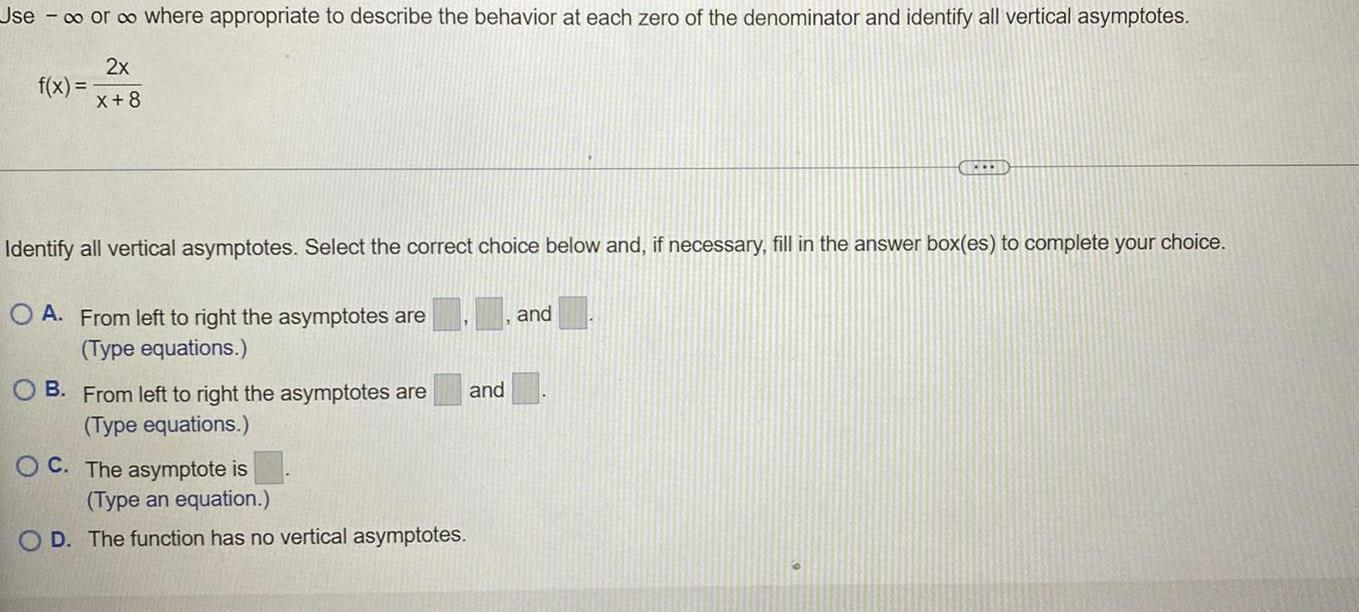Calculus
Limits & Continuity
Use - ∞o or ∞o where appropriate to describe the behavior at each zero of the denominator and identify all vertical asymptotes. f(x) = 2x/x+8 Identify all vertical asymptotes. Select the correct choice below and, if necessary, fill in the answer box(es) to complete your choice. A. From left to right the asymptotes are___,____and____ (Type equations.) B. From left to right the asymptotes are___and_____ (Type equations.) C. The asymptote is____ (Type an equation.) D. The function has no vertical asymptotes.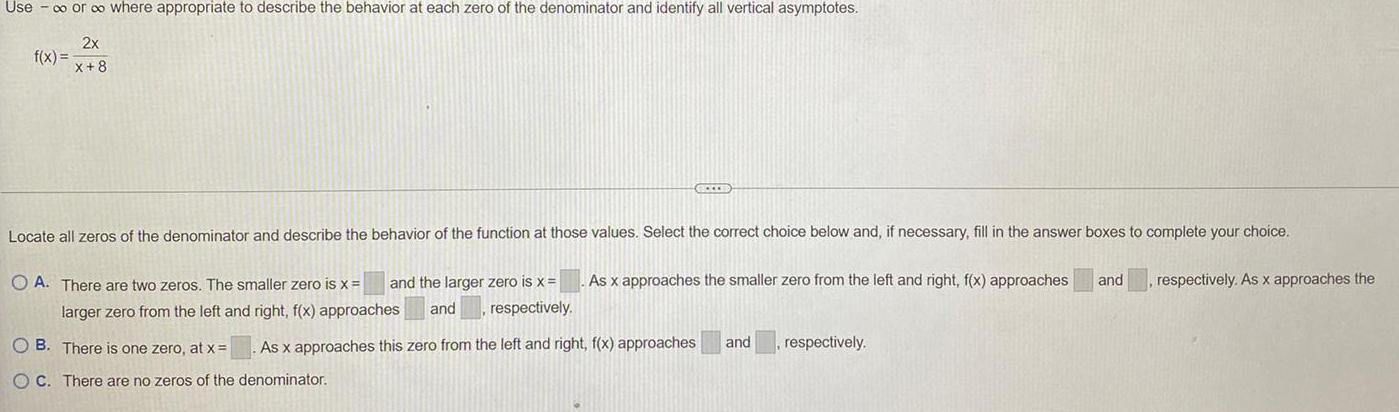Calculus
Limits & Continuity
Use - ∞ or ∞ where appropriate to describe the behavior at each zero of the denominator and identify all vertical asymptotes. f(x) = 2x/x+8 Locate all zeros of the denominator and describe the behavior of the function at those values. Select the correct choice below and, if necessary, fill in the answer boxes to complete your choice. As x approaches the smaller zero from the left and right, f(x) approaches and respectively. As x approaches the A. There are two zeros. The smaller zero is x= and the larger zero is x = larger zero from the left and right, f(x) approaches and respectively. B. There is one zero, at x = As x approaches this zero from the left and right, f(x) approaches and respectively. C. There are no zeros of the denominator.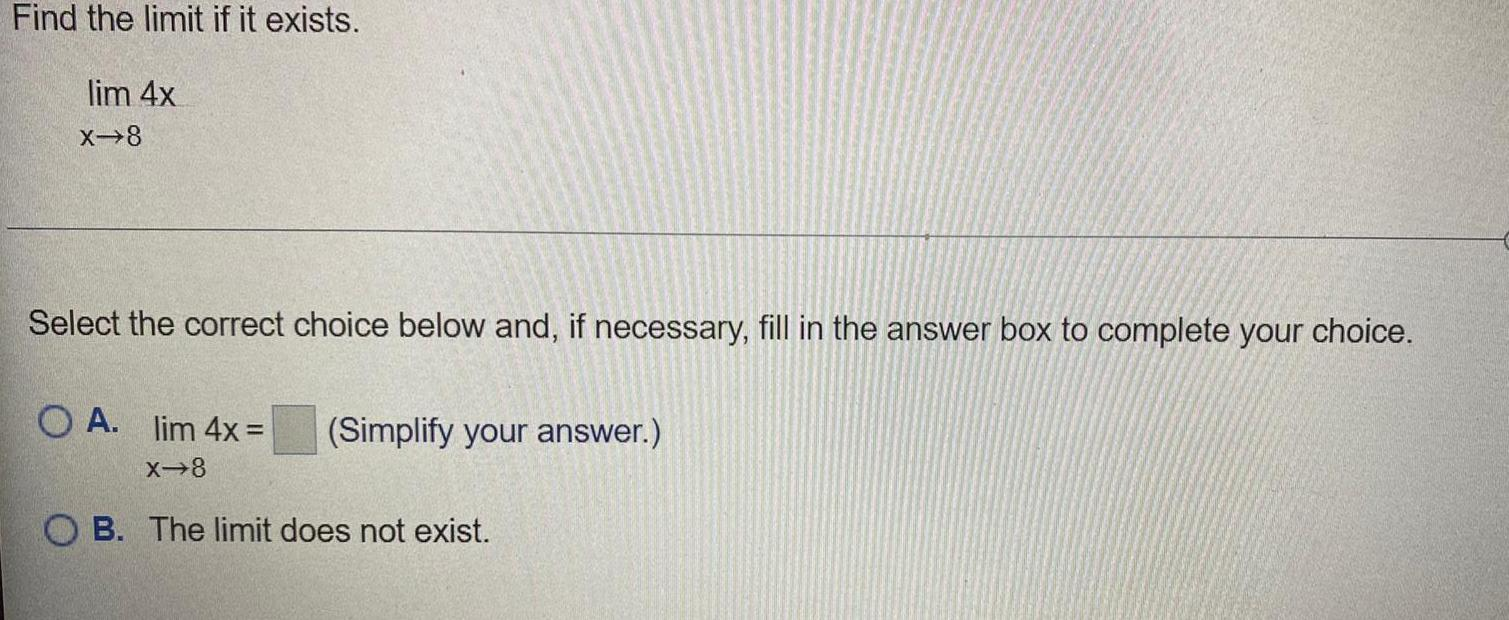Calculus
Limits & Continuity
Find the limit if it exists. lim 4x X→8 Select the correct choice below and, if necessary, fill in the answer box to complete your choice. A. lim 4x = X→8 (Simplify your answer.) B. The limit does not exist.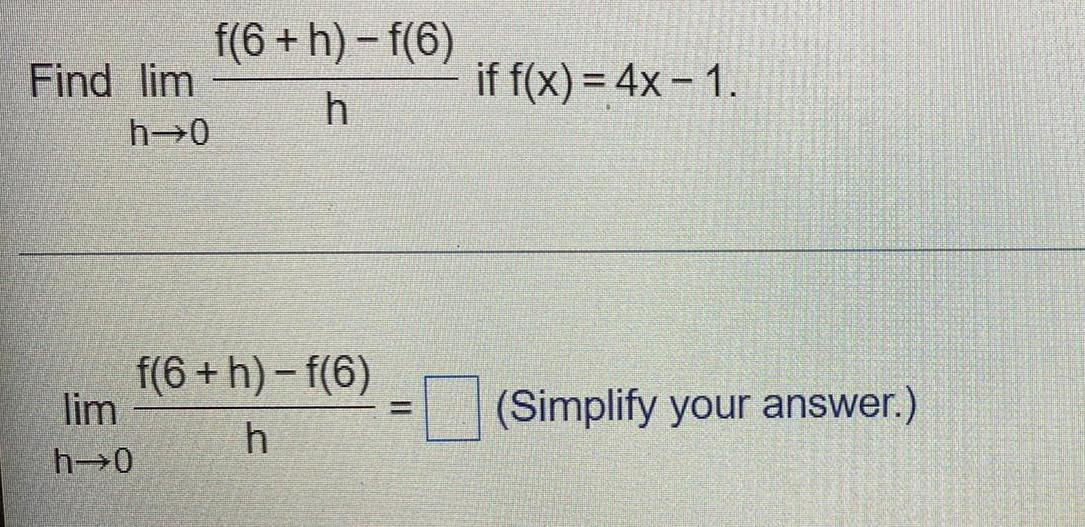Calculus
Limits & Continuity
Find lim f(6 +h)-f(6)/h if f(x) = 4x - 1. h→0 lim f(6 +h)-f(6)/h if f(x) = 4x - 1. h→0 (Simplify your answer.)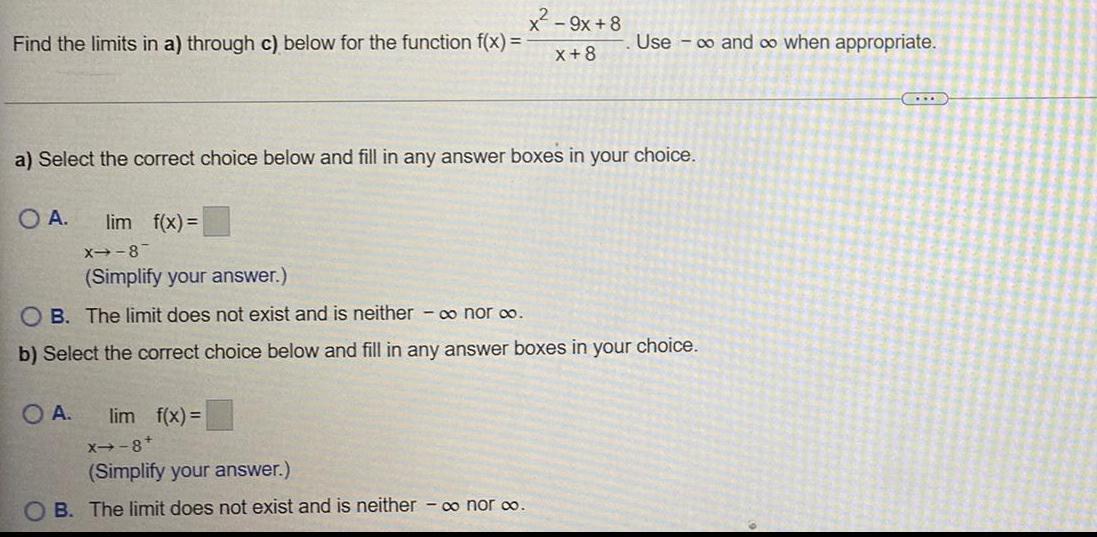Calculus
Limits & Continuity
Find the limits in a) through c) below for the function f(x) =x²-9x+8/ x+8 Use ∞ and ∞ when appropriate. a) Select the correct choice below and fill in any answer boxes in your choice. A. lim f(x)= X--8- B. The limit does not exist and is neither - ∞ nor ∞.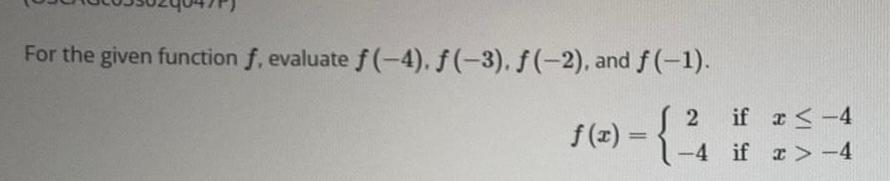Calculus
Limits & Continuity
For the given function f, evaluate f(-4), ƒ (-3), ƒ (-2), and f(-1). f(x) = 2 if x≤-4 -4 if x>-4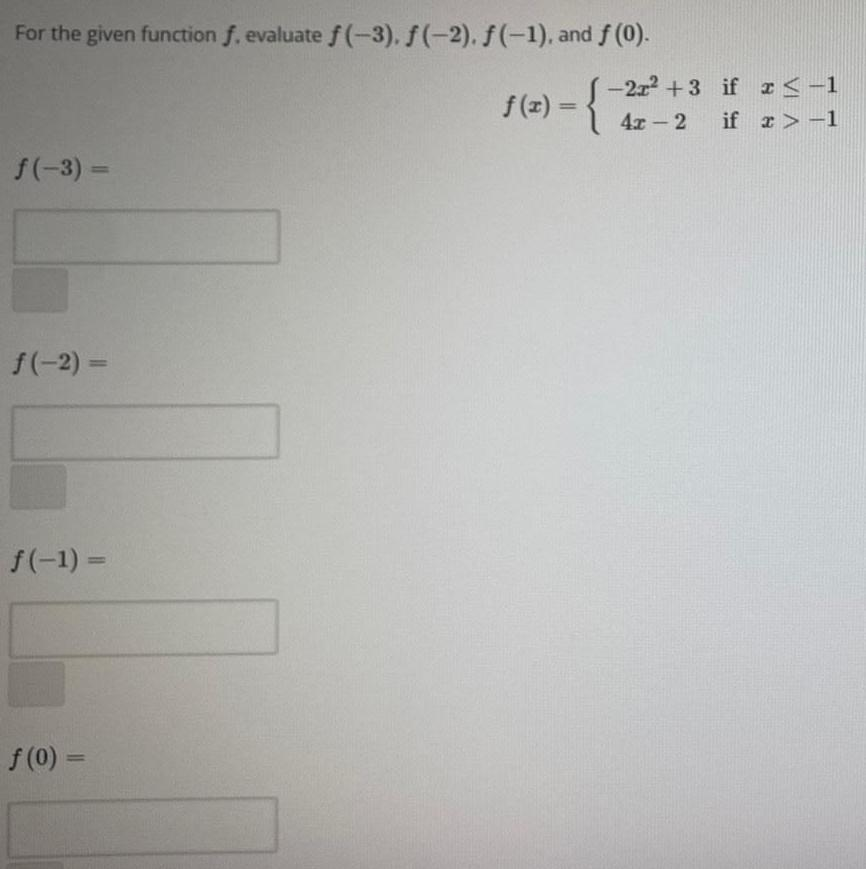Calculus
Limits & Continuity
For the given function f. evaluate f(-3), f(-2). f(-1), and f (0). f(x) =-2x²+3 if x < -1 4x-2 if x>-1 f(-3) = f(-2)= f(-1) = f(0) =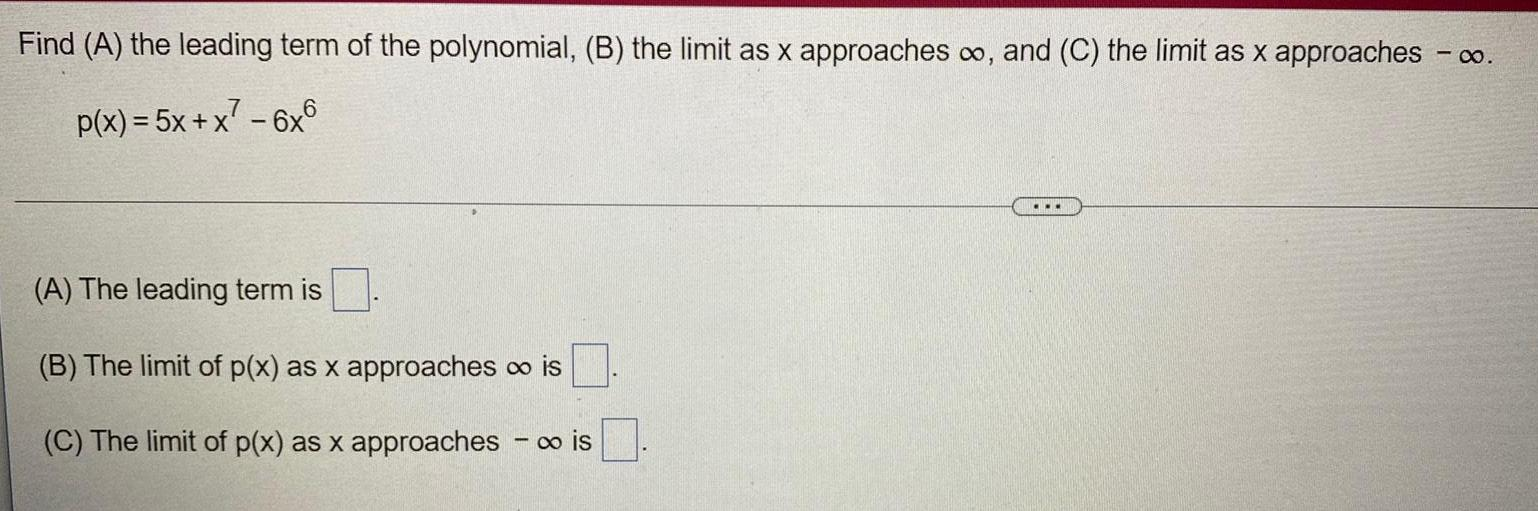Calculus
Limits & Continuity
Find (A) the leading term of the polynomial, (B) the limit as x approaches ∞o, and (C) the limit as x approaches - ∞ p(x) = 5x + x² - 6x6 (A) The leading term is (B) The limit of p(x) as x approaches ∞ is (C) The limit of p(x) as x approaches-∞ is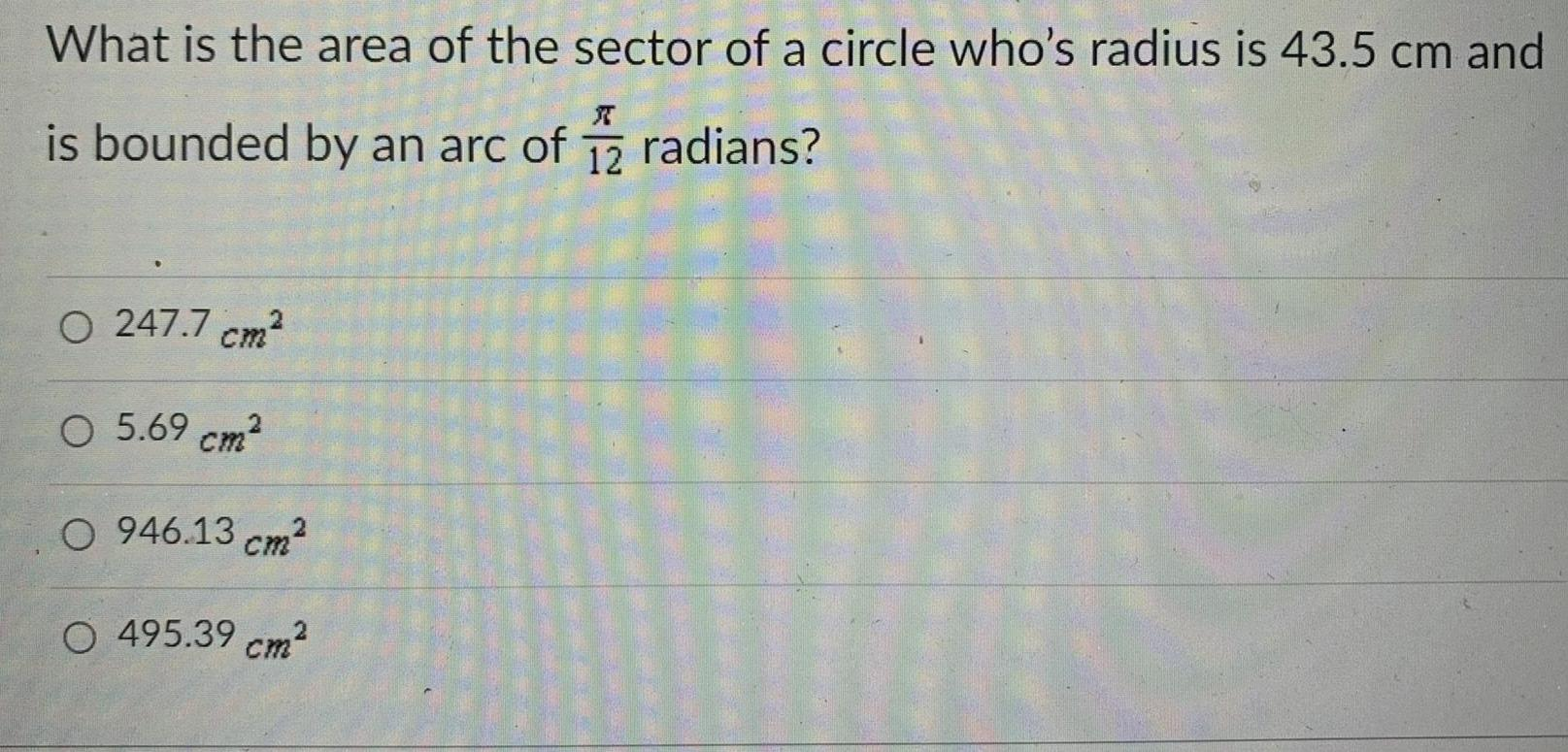Calculus
Limits & Continuity
What is the area of the sector of a circle who's radius is 43.5 cm and is bounded by an arc of π/12 radians? A. 247.7 cm2 B. 5.69 cm² C. 946.13 cm² D. 495.39 cm²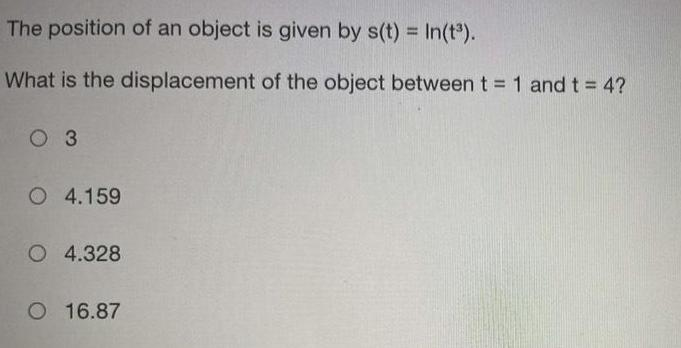Calculus
Limits & Continuity
The position of an object is given by s(t) = In(t³). What is the displacement of the object between t = 1 and t = 4? A. 3 B. 4.159 C. 4.328 D. 16.87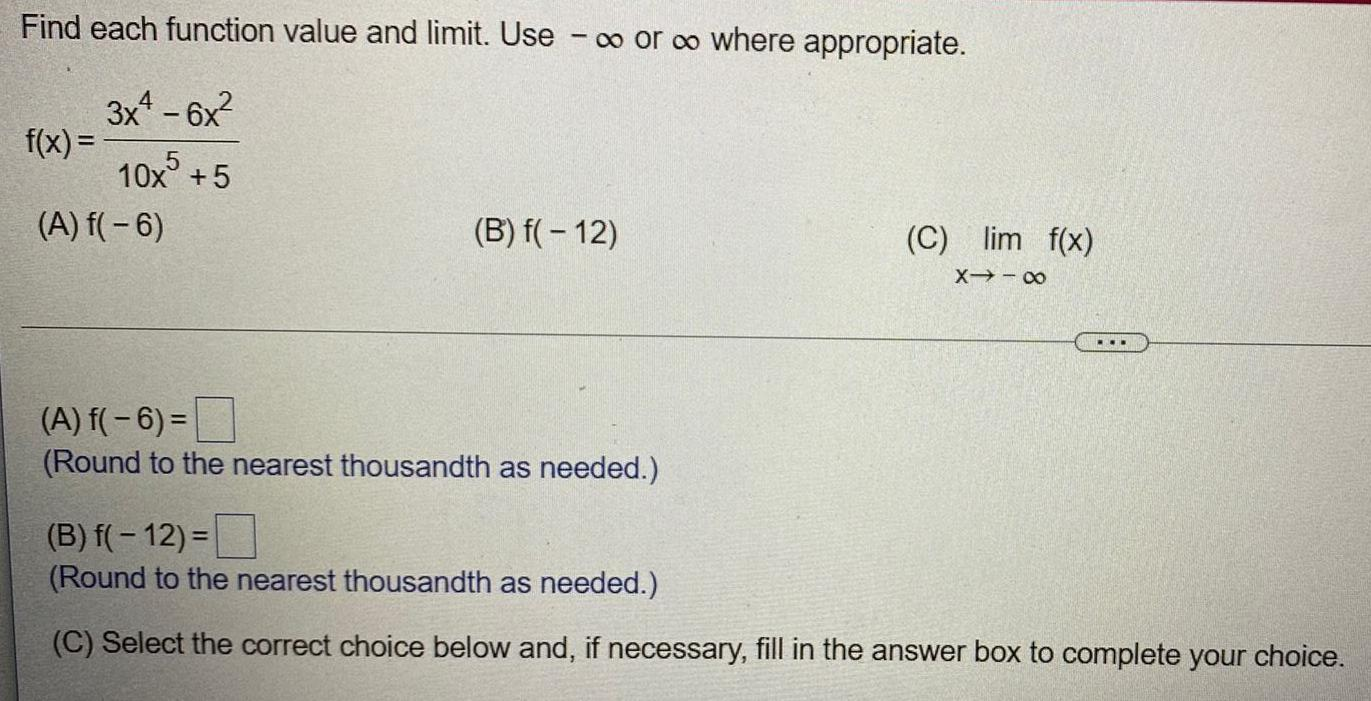Calculus
Limits & Continuity
Find each function value and limit. Use - ∞o or co where appropriate. f(x) = 3x² - 6x² / 10x⁵ + 5 (A) f(-6) (B) f(-12) (C) lim f(x) (A) f(-6)= (Round the nearest thousandth as needed.) (B) f(- 12) = (Round to the nearest thousandth as needed.) (C) Select the correct choice below and, if necessary, fill in the answer box to complete your choice.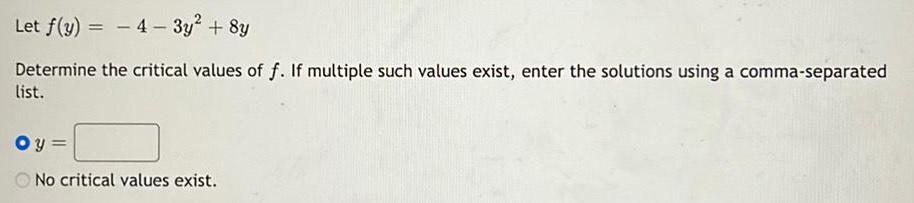Calculus
Limits & Continuity
Let f(y) = -4-3y² + 8y Determine the critical values of f. If multiple such values exist, enter the solutions using a comma-separated list.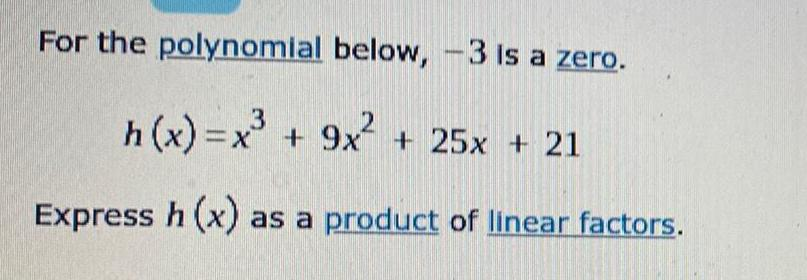Calculus
Limits & Continuity
For the polynomial below, -3 is a zero. h(x)=x³ + 9x² + 25x + 21 Express h (x) as a product of linear factors.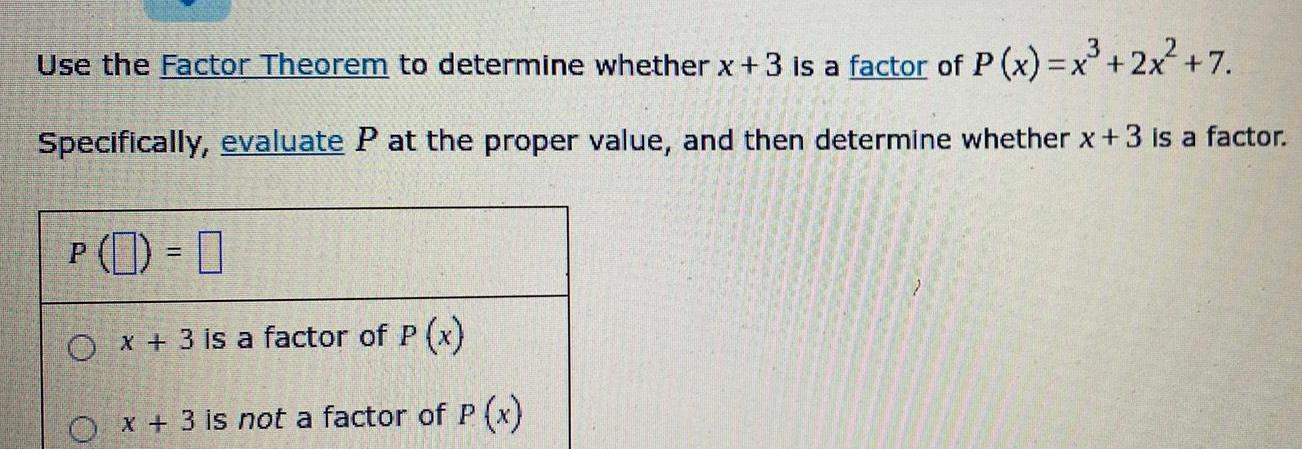Calculus
Limits & Continuity
Use the Factor Theorem to determine whether x + 3 is a factor of P(x) = x³ + 2x² +7. Specifically, evaluate P at the proper value, and then determine whether x + 3 is a factor.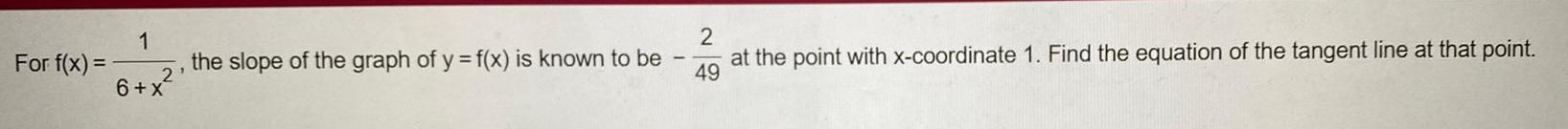Calculus
Limits & Continuity
For f(x) = 1/6+x², the slope of the graph of y = f(x) is known to be -2/49 at the point with x-coordinate 1. Find the equation of the tangent line at that point.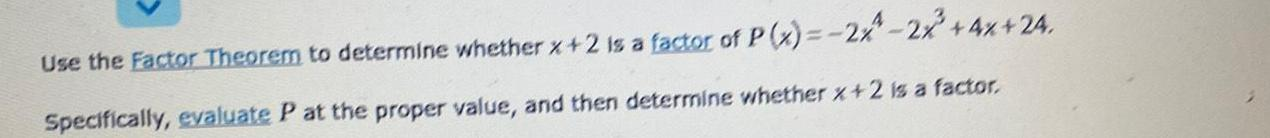Calculus
Limits & Continuity
Use the Factor Theorem to determine whether x +2 is a factor of P(x) = -2x⁴-2x³+4x+24. Specifically, evaluate P at the proper value, and then determine whether x + 2 is a factor.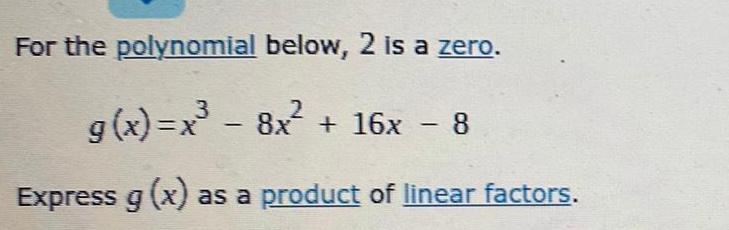Calculus
Limits & Continuity
For the polynomial below, 2 is a zero. g(x)=x³ - 8x² + 16x - 8 Express g (x) as a product of linear factors.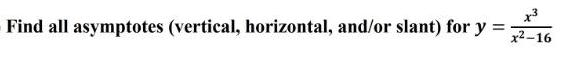Calculus
Limits & Continuity
Find all asymptotes (vertical, horizontal, and/or slant) for y = x³ / (x²-16 )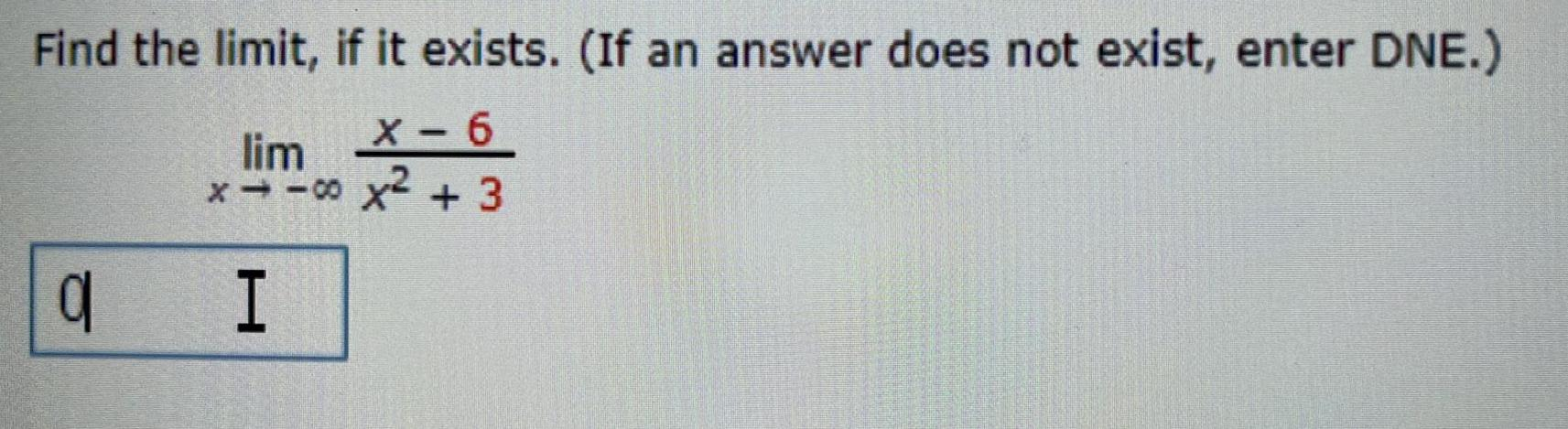Calculus
Limits & Continuity
Find the limit, if it exists. (If an answer does not exist, enter DNE.) lim x-6 / x² + 3 x→-∝